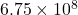## When you multiply (2.2500×10^3) x (3.00 x10^5) =675,000,000 report this answer to decimal using scientific notation

Question

When you multiply (2.2500×10^3) x (3.00 x10^5) =675,000,000 report this answer to decimal using scientific notation

in progress 0
5 months 2021-08-15T06:47:13+00:00 1 Answers 1 views 0Therefore, in scientific notation this will be represented as